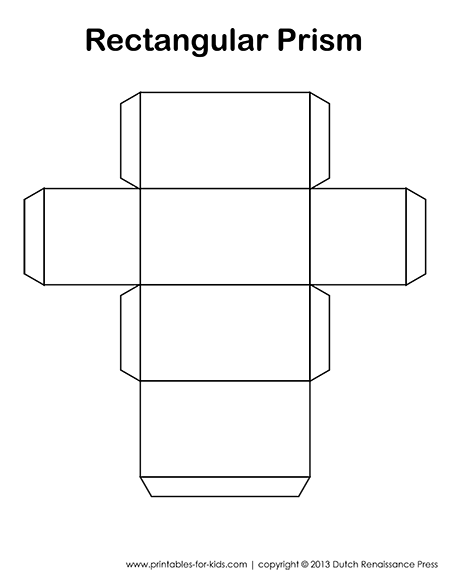# Rectangular Prism Template

If you’re learning about 3D shapes and geometry in school, here’s a free printable rectangular prism template. By building a rectangular prism crafts, students will be able to see how a 2D shape coverts into a 3D shape. When completed, they can turn the object, and count the vertices, faces, and edges.

To download the rectangular prism template PDF, click the image below. An 8.5 x 11 PDF will open in your browser. Once it loads, save it to your computer, or send it to your printer. Teachers, feel free to print multiple copies of these worksheets for your students.

##Surface Area FormulasThe surface area of a prism is equal to the sum of the areas of its faces. A rectangular prism is made up of 4 rectangle side faces and 2 rectangle cap faces.

Rectangular Prism Surface Area Formula:
2lw + 2lh + 2wh
Where:
l = the length of the rectangular prism
w = the width of the rectangular prism
h = the height of the rectangular prism

## Volume Formulas

Rectangular Prism Volume Formula:
Volume =lwh
Where:
l = the length of the rectangular prism
w = the width of the rectangular prism
h = the height of the rectangular prism

## Vertices, Faces, and Edges

A rectangular prism has 6 faces, 8 vertices, and 12 edges.

[jetpack-related-posts]